ReLU Activation Function and Its Variants

machine learningMl wizard

Activation functions in deep learning are the functions that decide the output from node or hidden layers from a given set of inputs in neural networks. There are many activation functions used in deep learning but among all of them rectified linear unit or ReLU is the widely used activation function in almost all deep learning neural networks.

# Table of Content

1. ReLU Activation Function
2. Dying ReLU Problem
3. Variants of ReLU
4. Leaky ReLU (LReLU)
5. Parametric ReLU (PReLU)
6. Exponential Linear Unit (ELU)
7. Scaled Exponential Linear Unit (SELU)
8. Conclusion

# ReLU Activation Function

The full form of the term ReLU is the rectified linear unit. ReLU is the activation function that is most widely used in neural network architectures.

The equation for the ReLU activation function is:

F(x) = max(0,x)

which tells that if the input x is less than zero then the output from the function will be zero and if the input given to the relu function is greater than zero then the output from the function will be the same as the input.Image Source

# Dying ReLU Problem

Due to the formula of the ReLU activation function, all the values less than zero will be zero after passing through the ReLU function. If the neural network is having a lot of negative values in the dataset then they all will be converted to zero after passing through the ReLU activation function and there will be zeros in the output which will make the neurons inactive. This type of scenario is called the dying ReLu problem in neural networks.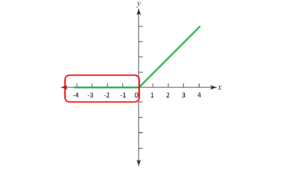Image Source

In the above image, we can see that all of the negative values passing through the ReLU activation function will be converted to zero and there will be a straight line in the graph of the function on the negative x-axis.

# Variants of ReLU

To solve the dying ReLU problems in the neural networks there are some other variants of the ReLU activation function that is developed, which do not face dying ReLU or dying neurons problem.

## 1. Leaky ReLU (LReLU)

The first and easiest variant of the ReLU activation function is leaky ReLU. there is only a little change in the formula of the normal ReLu activation function which solves the problem of dying ReLU.

The formula for the Leaky ReLU activation function is

F(x) = max(0.01x, x)

So according to the leaky ReLU if the input passes to the activation function are less than zero then the output value would be 0.01 times the input values and if the input passes to the activation function is greater than zero the output values will be equal to the input value.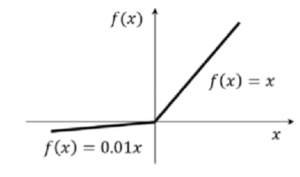Image Source

## 2. Parametric ReLU (PReLU)

Parametric ReLU is similar to the leaky ReLU activation function, but here instead of having a predetermined value for multiplying the input values with 0.01, we use the parameter “a” in parametric ReLU. The value of the “a” parameter will be decided by the neural network itself.

The formula for Parametric ReLU is

F(x) = max(ax, x)

which stated that if the input value given to the parametric RELU is less than zero then the output values will be equal to the input values multiplied with parameter “a” and if the input value if greater than zero then the output value will be the same as the input value.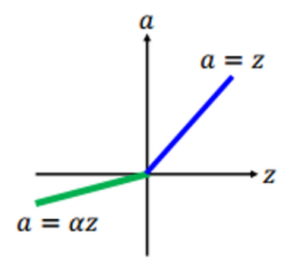Image Source

## 3. Exponential Linear Unit (ELU)

The exponential linear unit is a variant of ReLU activation which fixes some problems and gives some advantages compared to the normal ReLU activation function.

The formula for Exponential Linear Unit is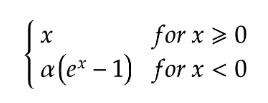Image Source

ELU activation function uses a log curve and due to this it does not gives a straight line for negative values, unlike leaky and parametric ReLU.

ELU activation functions also solve the dying ReLU problem and also the curve of the ELU becomes smooth slowly for negative values having high magnitude while normal Relu becomes smooth instantly.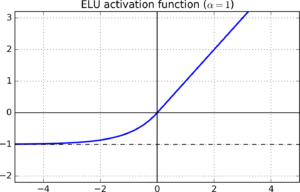Image Source

Higher computational powers are required while working with ELU compared to ReLU and there is a chance of exploding gradient problems while working with ELU.

## 4. Scaled Exponential Linear Unit (SELU)

This activation function is one of the latest activation functions in deep learning. The equation for this activation function just looks like the other equations. The equation for this activation function is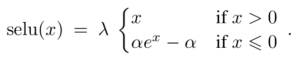Image Source

The equation states that, if the input value x is greater than zero, the output value becomes x multiplied by lambda λ term and If the input value x is less than or equal to zero then alpha is multiplied with the exponential of the x-value minus the alpha value, and then we multiply by the lambda value.

The calculated values for alpha and lambda are

Alpha = 1.6732632423543772848170429916717

Lambda = 1.0507009873554804934193349852946Image Source

The special case in the SELU activation function is that it is self normalizing activation function, which means that the input value will be passed through an activation function and the output from the function will be normalized by subtracting the mean from the value and diving it by its standard deviation. By doing this means centralizing will take place and the value of the mean will be zero and the standard deviation will be equal to 1.

# Conclusion

In this article, the ReLU activation function is discussed with its core mathematics behind it with dying ReLU problems and variants of ReLU like PReLU, LReLu, ELU, and SELU. Knowledge about the ReLU activation function and its variants will help one to understand its core intuition of it and will be able to differentiate between other variants of ReLU and their need of them.